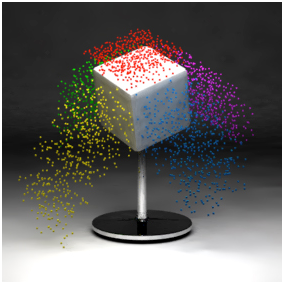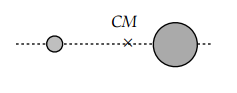Checkout JEE MAINS 2022 Question Paper Analysis : Checkout JEE MAINS 2022 Question Paper Analysis :

# System of Particles

An object of an ordinary size which we call a “macroscopic” system contains a huge number of atoms or molecules. It is out of the question to attempt to use the laws we have discussed for a single particle to describe separately each particle in such a system. There are nevertheless some relatively simple aspects of the behaviour of a macroscopic system that (as we will show) follow the basic laws for a particle. We will be able to show that in any multi-particle system each of the mechanical quantities relevant to the state of the system consists of two parts:• One part in which the system is treated as though it were a single particle (with the total mass of the system) located at a special point called the centre of mass. This part is often called the CM motion.
• Another part was describing the internal motion of the system, as seen by an observer located at (and moving with) the centre of mass.

## Systems of Particles and Rotational Motion – Video Lesson## Center Of Mass

We consider a system composed of N point particles, each labelled by a value of the index I which runs from 1 to N. Each particle has its mass mi and (at a particular time) is located at its particular place ri.

The centre of mass (CM) of the system is defined by the following position vector

###### $$\begin{array}{l}r_{CM}=\frac{1}{M}\sum_{i}m_{i}x_{i}\end{array}$$

Where M is the total mass of all the particles.

This vector locates a point in space — which may or may not be the position of any of the particles. It is the mass-weighted average position of the particles, being nearer to the more massive particles.

As a simple example, consider a system of only two particles, of masses m and 2m, separated by a distance. Choose the coordinate system so that the less massive particle is at the origin and the other is at x =  as shown in the drawing. Then we have m1 = m, m2 = 2m, x1 = 0 , x2 = .We find from the definition

$$\begin{array}{l}x_{cm}=\frac{1}{3m}\left ( m.0+2m \right )= \frac{2}{3}l\end{array}$$

The location is indicated on the drawing above.

As time goes on the position vectors of the particles ri generally change with time, so in general, the CM moves. The velocity of its motion is the time derivative of its position:

$$\begin{array}{l}v_{CM}=\frac{1}{M}\sum_{i=1}^{N}m_{1}v_{1}\end{array}$$

But the sum on the right side is just the total (linear) momentum of all the particles. Solving for this, we find an important result:

###### $$\begin{array}{l}P_{tot}=Mv_{CM}\end{array}$$

This is just like the formula for the momentum of a single particle, so we see that: The total momentum of a system is the same as if all the particles were located together at the CM and moving with its velocity.

## Frequently Asked Questions on System of Particles

### What is the significance of the centre of mass of the system of particles?

Centre of mass of the system of particles helps in describing the behaviour of a macroscopic body in terms of the laws developed for microscopic particles.

### What do you understand by the centre of mass of a system of particles?

The point in the system which will move in the same way that a single particle (of mass equal to the sum of the masses of all the particles in the system) would move when subject to external force is called the centre of mass of the system.

### What is the centre of mass of a system of particles?

A system’s or a body’s centre of mass is a point where all of the system’s mass is supposed to be concentrated, and this point like mass has the same kind of translational motion as the system as a whole if the same net external force operates on this point like mass as on the system as a whole.

Test your Knowledge on System of particles